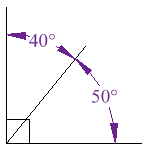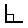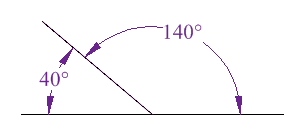# Complementary and Supplementary Angle

1. What is a complementary and supplementary angle?
2. How do you find a complementary & supplementary angles?

Before we define what we mean by complementary and supplementary angles, here is an important reminder about adjacent angles
Adjacent angles: Angles that share a vertex and a common side.
However angles do not have to be adjacent to be complementary or supplementary.

## Complementary Angles

» Two angles are complementary if the sum of their angles equals 900
» If one angle is known, its complementary angle can be found by subtracting the measure of its angle from 900.
Example: What is the complementary angle of 400Solution:  90- 400  =  500

» These two angles (40° and 50°) are Complementary Angles, because they add up to 90°:
»Notice that together they make a right angle.

## Supplementary Angles

»Two angles are supplementary if the sum of their angles equals 1800.
»If one angle is known, its supplementary angle can be found by subtracting the measure of its angle from 1800.
Example: What is the supplementary angle of 1400?Solution: 1800 - 1400  =  400
»These two angles (140° and 40°) are Supplementary Angles, because they add up to 180°:
»Notice that together they make a straight angle.Thanks for reading Complementary and Supplementary Angle

←Previous
« Prev Post
Next→
Next Post »

Video Lectures